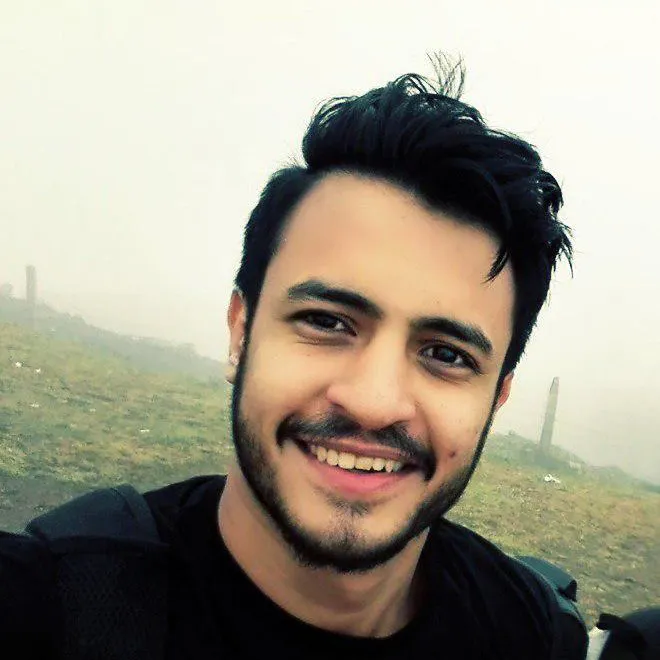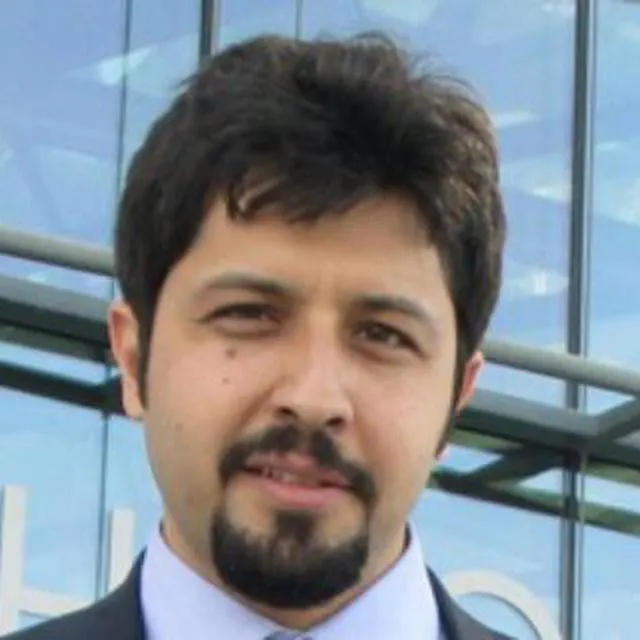Published © GPL3+

# Goal-Line Technology

In association football, Goal-Line Technology is the use of electronic aid to determine if a goal has been scored or not.

BeginnerWork in progress8 hours3,985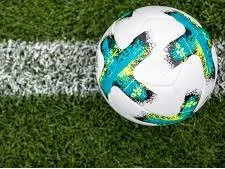## Things used in this project

### Hardware componentsRaspberry Pi 3 Model B
×1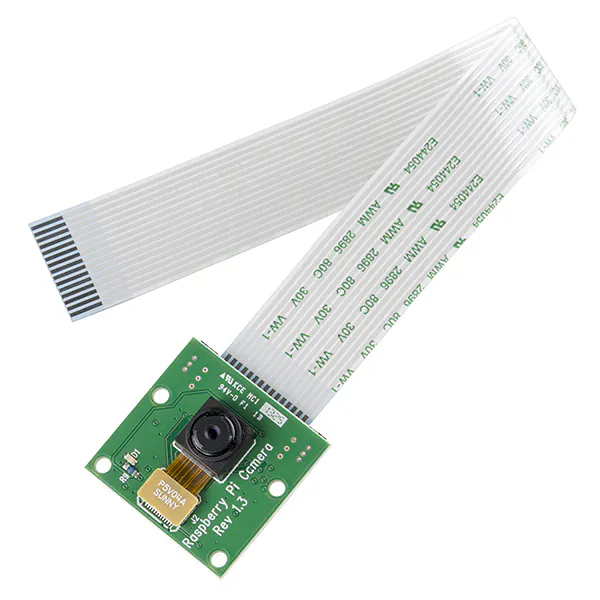Raspberry Pi Camera Module
×1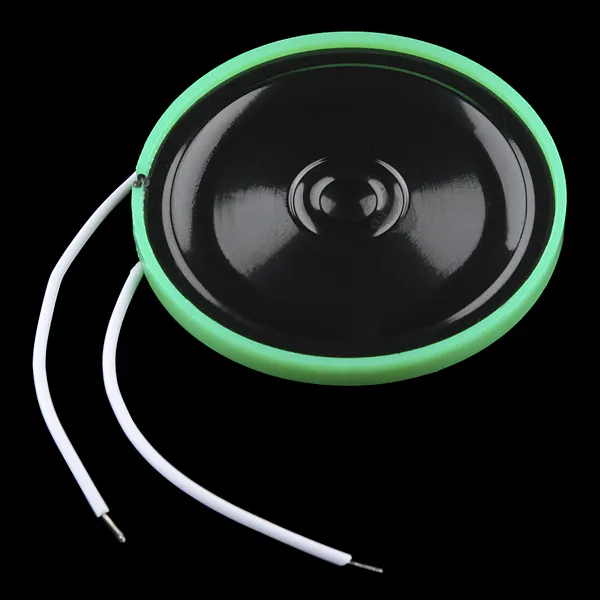Speaker: 0.25W, 8 ohms
×1

### Software apps and online services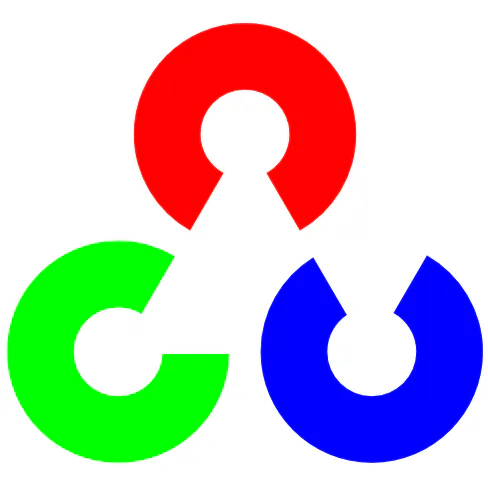OpenCV

Read more

## Schematics

### Goal Decision System in one pic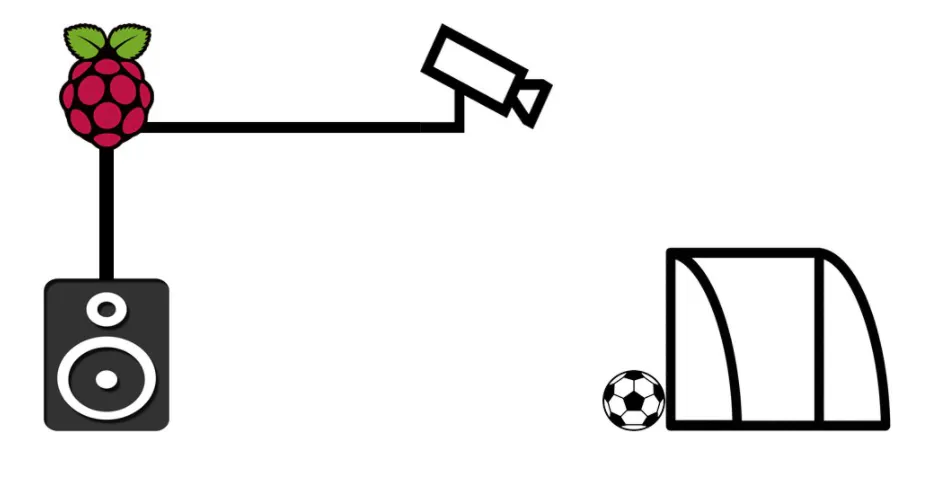## Code

### Ball Detection

Python
```# python dynamic_color_tracking.py --filter HSV --webcam

import cv2
import argparse
import numpy as np

def callback(value):
pass

def setup_trackbars(range_filter):
cv2.namedWindow("Trackbars", 0)

for i in ["MIN", "MAX"]:
v = 0 if i == "MIN" else 255

for j in range_filter:
cv2.createTrackbar("%s_%s" % (j, i), "Trackbars", v, 255, callback)

def get_arguments():
ap = argparse.ArgumentParser()
ap.add_argument('-f', '--filter', required=True,
help='Range filter. RGB or HSV')
ap.add_argument('-w', '--webcam', required=False,
help='Use webcam', action='store_true')
args = vars(ap.parse_args())

if not args['filter'].upper() in ['RGB', 'HSV']:
ap.error("Please speciy a correct filter.")

return args

def get_trackbar_values(range_filter):
values = []

for i in ["MIN", "MAX"]:
for j in range_filter:
v = cv2.getTrackbarPos("%s_%s" % (j, i), "Trackbars")
values.append(v)
return values

def main():
args = get_arguments()

range_filter = args['filter'].upper()

camera = cv2.VideoCapture(0)

setup_trackbars(range_filter)

while True:
if args['webcam']:
ret, image = camera.read()

if not ret:
break

if range_filter == 'RGB':
frame_to_thresh = image.copy()
else:
frame_to_thresh = cv2.cvtColor(image, cv2.COLOR_BGR2HSV)

v1_min, v2_min, v3_min, v1_max, v2_max, v3_max = get_trackbar_values(range_filter)

thresh = cv2.inRange(frame_to_thresh, (v1_min, v2_min, v3_min), (v1_max, v2_max, v3_max))

kernel = np.ones((5, 5), np.uint8)
mask = cv2.morphologyEx(thresh, cv2.MORPH_OPEN, kernel)
mask = cv2.morphologyEx(mask, cv2.MORPH_CLOSE, kernel)

# find contours in the mask and initialize the current
# (x, y) center of the ball
cnts = cv2.findContours(mask.copy(), cv2.RETR_EXTERNAL, cv2.CHAIN_APPROX_SIMPLE)[-2]
center = None

# only proceed if at least one contour was found
if len(cnts) > 0:
# find the largest contour in the mask, then use
# it to compute the minimum enclosing circle and
# centroid
c = max(cnts, key=cv2.contourArea)
((x, y), radius) = cv2.minEnclosingCircle(c)
M = cv2.moments(c)
center = (int(M["m10"] / M["m00"]), int(M["m01"] / M["m00"]))

# only proceed if the radius meets a minimum size
if radius > 10:
# draw the circle and centroid on the frame,
# then update the list of tracked points

cv2.circle(image, (int(x), int(y)), int(radius), (0, 255, 255), 2)
#  cv2.circle(image, center, 3, (0, 0, 255), -1)
#  cv2.putText(image, "centroid", (center + 10, center), cv2.FONT_HERSHEY_SIMPLEX, 0.4, (0, 0, 255),
#              1)
#  cv2.putText(image, "(" + str(center) + "," + str(center) + ")", (center + 10, center + 15),
#             cv2.FONT_HERSHEY_SIMPLEX, 0.4, (0, 0, 255), 1)

# show the frame to our screen
if x < 200:
cv2.putText(image, "GOOOAALLLL!! ", (50, 50), cv2.FONT_HERSHEY_COMPLEX_SMALL, .7, (0, 255, 0))
else:
cv2.putText(image, "NO GOAL", (50, 50), cv2.FONT_HERSHEY_COMPLEX_SMALL, .7, (0, 0, 255))
cv2.line(image, (200, 0), (200, 511), (255, 0, 0), 5)
cv2.imshow("Original", image)
cv2.imshow("Thresh", thresh)
cv2.imshow("Mask", mask)

if cv2.waitKey(1) & 0xFF is ord('q'):
break

if __name__ == '__main__':
main()
```

### Ball Detection

Python
using this code you can detect a ball and say that whether it crossed the line or not
```# python dynamic_color_tracking.py --filter HSV --webcam

import cv2
import argparse
import numpy as np

def callback(value):
pass

def setup_trackbars(range_filter):
cv2.namedWindow("Trackbars", 0)

for i in ["MIN", "MAX"]:
v = 0 if i == "MIN" else 255

for j in range_filter:
cv2.createTrackbar("%s_%s" % (j, i), "Trackbars", v, 255, callback)

def get_arguments():
ap = argparse.ArgumentParser()
ap.add_argument('-f', '--filter', required=True,
help='Range filter. RGB or HSV')
ap.add_argument('-w', '--webcam', required=False,
help='Use webcam', action='store_true')
args = vars(ap.parse_args())

if not args['filter'].upper() in ['RGB', 'HSV']:
ap.error("Please speciy a correct filter.")

return args

def get_trackbar_values(range_filter):
values = []

for i in ["MIN", "MAX"]:
for j in range_filter:
v = cv2.getTrackbarPos("%s_%s" % (j, i), "Trackbars")
values.append(v)
return values

def main():
args = get_arguments()

range_filter = args['filter'].upper()

camera = cv2.VideoCapture(0)

setup_trackbars(range_filter)

while True:
if args['webcam']:
ret, image = camera.read()

if not ret:
break

if range_filter == 'RGB':
frame_to_thresh = image.copy()
else:
frame_to_thresh = cv2.cvtColor(image, cv2.COLOR_BGR2HSV)

v1_min, v2_min, v3_min, v1_max, v2_max, v3_max = get_trackbar_values(range_filter)

thresh = cv2.inRange(frame_to_thresh, (v1_min, v2_min, v3_min), (v1_max, v2_max, v3_max))

kernel = np.ones((5, 5), np.uint8)
mask = cv2.morphologyEx(thresh, cv2.MORPH_OPEN, kernel)
mask = cv2.morphologyEx(mask, cv2.MORPH_CLOSE, kernel)

# find contours in the mask and initialize the current
# (x, y) center of the ball
cnts = cv2.findContours(mask.copy(), cv2.RETR_EXTERNAL, cv2.CHAIN_APPROX_SIMPLE)[-2]
center = None

# only proceed if at least one contour was found
if len(cnts) > 0:
# find the largest contour in the mask, then use
# it to compute the minimum enclosing circle and
# centroid
c = max(cnts, key=cv2.contourArea)
((x, y), radius) = cv2.minEnclosingCircle(c)
M = cv2.moments(c)
center = (int(M["m10"] / M["m00"]), int(M["m01"] / M["m00"]))

# only proceed if the radius meets a minimum size
if radius > 10:
# draw the circle and centroid on the frame,
# then update the list of tracked points

cv2.circle(image, (int(x), int(y)), int(radius), (0, 255, 255), 2)
#  cv2.circle(image, center, 3, (0, 0, 255), -1)
#  cv2.putText(image, "centroid", (center + 10, center), cv2.FONT_HERSHEY_SIMPLEX, 0.4, (0, 0, 255),
#              1)
#  cv2.putText(image, "(" + str(center) + "," + str(center) + ")", (center + 10, center + 15),
#             cv2.FONT_HERSHEY_SIMPLEX, 0.4, (0, 0, 255), 1)

# show the frame to our screen
if x < 200:
cv2.putText(image, "GOOOAALLLL!! ", (50, 50), cv2.FONT_HERSHEY_COMPLEX_SMALL, .7, (0, 255, 0))
else:
cv2.putText(image, "NO GOAL", (50, 50), cv2.FONT_HERSHEY_COMPLEX_SMALL, .7, (0, 0, 255))
cv2.line(image, (200, 0), (200, 511), (255, 0, 0), 5)
cv2.imshow("Original", image)
cv2.imshow("Thresh", thresh)
cv2.imshow("Mask", mask)

if cv2.waitKey(1) & 0xFF is ord('q'):
break

if __name__ == '__main__':
main()
```

## Credits

### Vahid Jafari

1 project • 6 followers
" Vahid Jafari " is a computer student at Hamedan University of Technology (HUT). He's is really interested in the computer and technology.

### Amin fadaee

1 project • 2 followers
Bs in IT engineering at Hamedan University of Technology Ms in Computer HW engineering at Sharif University of Technology

### Ehsan Aerabi

18 projects • 57 followers
Researcher on IoT and Embedded Systems